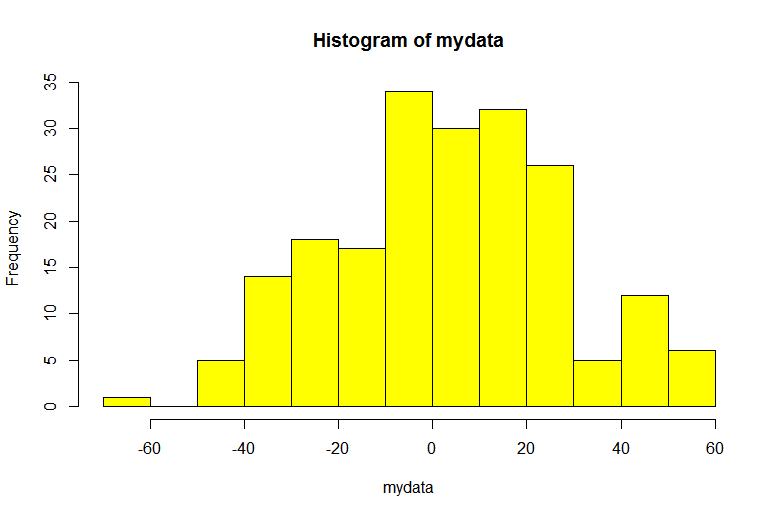# And Data Asks, “Do I Look Normal to You?”Normality tests for data science. Here we have put the null hypothesis as data is normally distributed, typically it depicts that if data is normal then we will not take any action and we will proceed for building a prediction model.

It is very important as a data analyst or data scientist, one has to assess a sample data at first, source of sample data can be primary or secondary but it is very important to analyze the nature of the data.

Let’s put this into a hypothesis as follows,

NULL Hypothesis: On average, sample data distribution is normal

*ALTERNATE Hypothesis: *On average, sample data distribution is not normal

What does it mean? Hypothesis Formulations

Let us see, here we have put the null hypothesis as data is normally distributed, typically it depicts that if data is normal then we will not take any action and we will proceed for building a prediction model.

In case of alternate hypothesis, it depicts that if data is not normally distributed then we will have to take any action before proceeding for building a prediction model, typical action as a data scientist can be the normalization of a data once this corrective action is taken then only he or she will proceed for building a prediction model.

Strategies to test normality of data

Now in order to test whether a sample data is normal or not, we will decide strategies at first that are as follows,

## Data Visualization Methods:

These will visualize the nature of data but not able to quantify the same.

1. Plotting a histogram, graphical distribution of frequencies within the range
2. Plotting Q-Q plot, a graphical method for comparing of probability distributions.

## Exploratory Data Analysis is a significant part of Data Science

Data science is omnipresent to advanced statistical and machine learning methods. For whatever length of time that there is data to analyse, the need to investigate is obvious.

## Exploratory Data Analysis is a significant part of Data Science

You will discover Exploratory Data Analysis (EDA), the techniques and tactics that you can use, and why you should be performing EDA on your next problem.

## Data Cleaning in R for Data Science

A data scientist/analyst in the making needs to format and clean data before being able to perform any kind of exploratory data analysis.

## Data Analysis and Visualization of scraped data from IMDb with R

TV Series that Geeks (and not so geeks) love

## Beginner’s Guide: Exploratory Data Analysis in R

This article is a guide for people classified under the tag of ‘Data Science infants.’ I believe both Python and R are great languages, and what matters most is the Story you tell from your data.